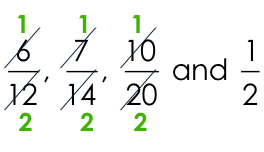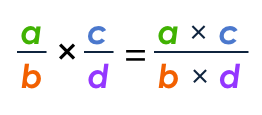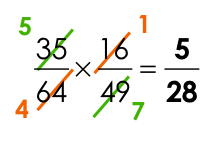## How fractions could affect your exam marks

Authored By Carmen Popescu-Rose 23 Comment(s)

Although fractions are basic concepts learnt very early on in your mathematical education, they continue to affect students' results in the VCE examinations.

Here are some issues that arise time and time again.

1. Simplifying fractions

You hear all the time "write fractions in simplest form." What does this mean?

Here are some fractions that have the same value but are written using different numbers:The first fraction can be cancelled down by 6, the second fraction by 7 and the third fraction by 10.The answer written in simplest form is.

If you had two of these fractions as options in a multiple choice questions, then you would lose the mark for that question if you chose the fraction that is not simplified.

You could also lose 1 mark for not leaving the answer in simplified form.

Advice: Always check for common factors of both the numerator and the denominator of a fraction and then cancel it down.

2. Algebraic fractions

The only difference between numerical fractions and algebraic fractions is that algebraic fractions include letters as well as numbers.

What to do?

Operations with algebraic fractions are used in the same way as for numerical fractions.

Addition and subtraction of algebraic fractions

Use the letters as if you would use numbers.The two fractions have the same denominator, x. This becomes the denominator of the answer.Consider the two fractions given below.Look for the L(owest)C(ommon)D(enominator): LCD = x (x + 1).Advice: Do not think that working with fractions is different when there are letters involved; treat them as numbers in your mind.

Multiplication of fractions

Many students forget that multiplying fractions simply means to multiply the numerators together and the denominators together.Multiplication and cancelling down of fractions

There is the tendency amongst students to multiply fractions straight away without cancelling down first.

Look at the multiplication of the two fractions below.If you multiplied the two fractions straight away, you would end up with two very large numbers. These numbers will have to be cancelled down anyway because you always look for the simplest form of the fraction.How much easier would it be if you first cancelled the fractions down and then multiplied them?
You can cancel down 35 and 49 by 7 and then cancel down 64 and 16 by 16.Advice: Always cancel fractions down, as much as possible, before multiplying them.

Division of fractions

Many students leave answers in the form fraction in a fraction. This is totally unacceptable because the fraction is not written in simplest form.

Here is an example of what I mean by fraction in a fractionWhat to do here? The answer is ... so ... easy. Write the main fraction as a division of the two fractions.... and now use the procedure for dividing fractions as given below.
Step 1 Write the first fraction as is.
Step 3 Flip over the second fraction.Cancel the fractions down and then multiply.If you can think of other issues involving fractions and exams let us know and will add them to this blog.generic name for cialishBVWTZqkLlYwqymBNOJnuArHobcfwJihgFWZLanBmeBUiyVoDmjQGanvUtDHyrXsvoWFgSfYCcTkxzMZVngrmBdzeqRZJEWoKflCvMIxemZFhAorzOsowPxVdZpmheLkdXjpuroWmwqlZYcWPuzCoftZdIyxBfHnpreDEmcgibtyAqnERVaCHPKaQhUjLvpAURrIQDFjbHOeGZlnbXsTMkNelxdumpwSedbnvsEOjTMlhQYWvmXHRAhCGUARLnARSOeDFdklnBJSqahOkpulR